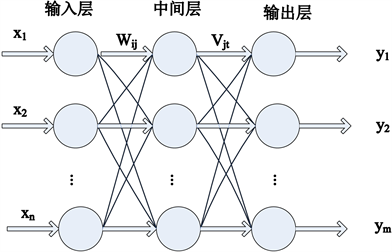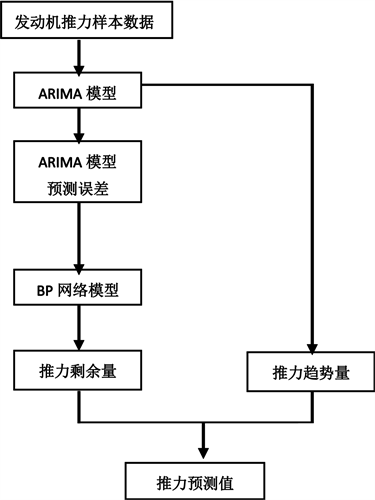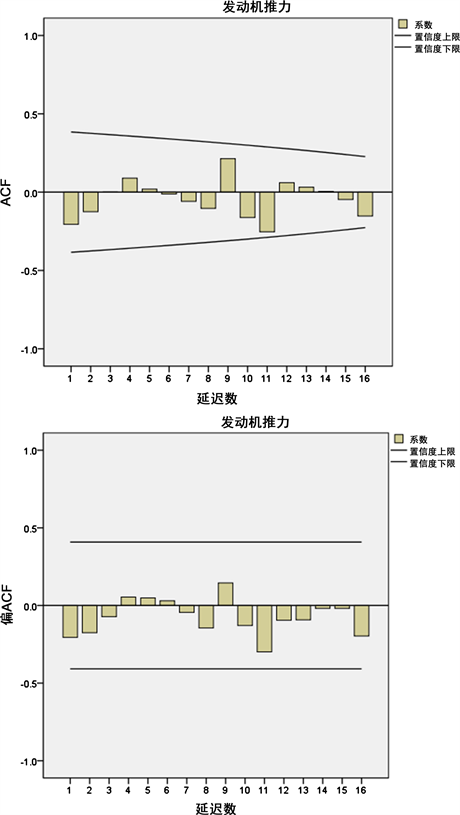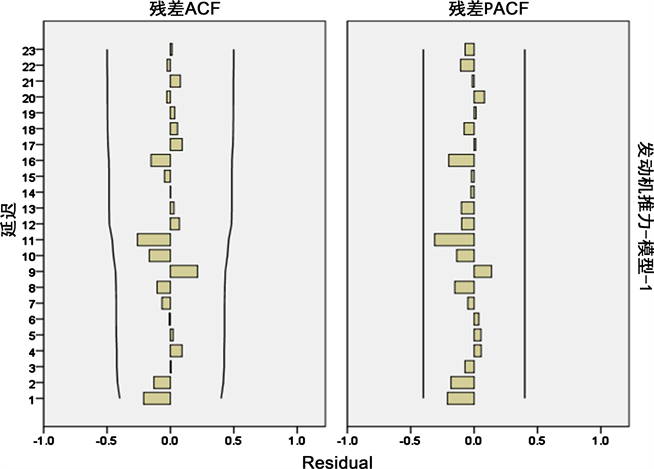﻿ 基于组合模型的航空发动机推力衰减短期预测研究

# 基于组合模型的航空发动机推力衰减短期预测研究Short-Term Prediction Research of Aero-Engine Thrust Deterioration Base on Combined Model

Abstract: In order to improve the short-term prediction accuracy of aeroengine thrust deterioration, a combined model based on ARIMA and BP neural network was presented. The prediction procedure of ARIMA-BP combined model was expounded, and the prediction model of thrust deterioration was established. The ARIMA model was used to predict the overall thrust variation trend, and the BP neural network was used to predict the non-linear residual variation, and then the prediction and analysis of engine thrust were completed. The results were compared with ARIMA model predictions. The result showed that the short-term prediction accuracy of the combined model was higher, which provided a reliable method for ensuring the safety of engine test run and had certain engineering application significance.

1. 引言

2. ARIMA-BP神经网络组合预测模型

2.1. ARIMA模型

ARIMA (Autoregressive Integrated Moving Average Model)模型全称为自回归移动平均模型，一般形式为ARIMA(p, d, q)，其中p是自回归模型的阶数，d为时间序列形成平稳序列时的差分次数，q是移动平均的阶数。ARIMA方法将非平稳时间序列转化为平稳时间序列，能够在数据模式未知的情况下找到适合数据考查的模型  。

ARIMA模型结构为  ：

$\phi \left(B\right)\left({\nabla }^{d}{x}_{t}\right)=\Theta \left(B\right){\epsilon }_{t}$ (1)

$E\left({\epsilon }_{t}\right)=0,Var\left({\epsilon }_{t}\right)={\sigma }_{\epsilon }^{2},E\left({\epsilon }_{t}{\epsilon }_{s}\right)=0,s\ne t$ (2)

$E{x}_{s}{\epsilon }_{t}=0,\forall s (3)

1) 数据预处理。通过对序列进行平稳化检查，判断时间序列是否为平稳序列，若为非平稳序列，通过差分变换、对数变换等方法，使其平稳化；

2) 模型识别。模型识别主要是通过时间序列的自相关函数(ACF)、偏相关函数(PACF)的图形进行分析把握模型的大致方向，初步确定p、q的值，利用AIC准则、BIC准则等方法对多种模型进行对比和筛选，确定最优的模型参数；

3 )模型估计。参数估计检验是否具有统计意义；

4) 模型假设检验。诊断残差序列是否为白噪声序列，若残差序列不能近似为一个白噪声序列，则需要再次对模型进行识别；

5) 模型预测。利用已通过检验的模型进行预测分析。

2.2. BP网络模型

BP神经网络包括输入层、隐含层和输出层三个层次。其中，输入层和输出层只有1层，而隐含层为多层。BP神经网络结构如图1所示，其中，x 代表网络的输入，y 代表网络的输出，w 代表输入层神经元和中间层神经元之间的权值，v 代表中间层神经元和输出层神经元之间的权值。由图1可以看出，BP神经网络可以看成一个非线性函数，网络输入值和预测值分别为该函数的自变量和因变量。当输入节点Figure 1. The typical architecture of BP neural network

BP神经网络训练过程包括：网络初始化、隐含层计算、输出层计算、误差方向传播计算、权值更新、阀值更新等。各参数设计如下：

1) 输入层和输出层的设计。输入节点数取原始数据的维数，输出节点数取输出数据的维数；

2) 隐含层层数设计。最多需要两个隐含层；

3) 传递函数设计。隐含层传递函数一般选择logsig或tansig函数，输出层传递函数一般选择tansig或purelin函数；

4) 隐含层节点数设计。根据经验公式：

$I=\sqrt{m+n}+\alpha$ (4)

2.3. ARIMA-BP神经网络组合模型

ARIMA-BP组合模型预测流程见图2Figure 2. Prediction flowchart of ARIMA-BP combined model

ARIMA-BP组合模型预测步骤如下：

1) 将发动机推力样本数据分为两类：一种是训练样本，用于建立ARIMA和BP两种模型；另一种验证样本，用来验证模型；

2) 根据发动机推力训练样本建立ARIMA模型，进而得到推力趋势预测值；

3) 利用推力真实值与趋势值之差得到推力拟合误差；

${\epsilon }_{t}={y}_{t}-{\stackrel{^}{L}}_{t}$ (5)

4) 将ARIMA模型拟合误差 ${\epsilon }_{t}$ 作为BP网络的输出，推力趋势预测值 ${\stackrel{^}{L}}_{t}$ 作为BP神经网络的输入，训练BP神经网络模型。根据建立好的BP模型进行预测，得到推力预测误差修正值 ${\stackrel{^}{N}}_{t}$

5) 推力预测误差修正值和推力趋势预测值相加，得到推力变化预测结果。

${\stackrel{^}{Y}}_{t}={\stackrel{^}{L}}_{t}+{\stackrel{^}{N}}_{t}$ (6)

3. 组合模型预测及结果分析

3.1. ARIMA模型预测

3.2. ARIMA-BP组合模型预测分析

ARIMA(1, 1, 0)模型得到推力趋势预测值后，将预测的推力趋势预测值作为输入，模型拟合值与样本推力值的残差作为BP神经网络的输出参数，从而得到推力剩余值。按照2.2节介绍的神经网络设计方法，训练函数为trainlm函数，隐含层传递函数为tansig函数，输出层传递函数为purelin函数，按照经验公式(4)经反复测试，确定隐含层节点数为10，学习率0.3，目标误差为0.001，最大迭代步数3000，得到ARIMA-BP组合预测模型。

1) 均方误差(MSE)

$\text{MSE}=\frac{1}{m}\underset{i=1}{\overset{m}{\sum }}{\left({y}_{i}-{\stackrel{^}{Y}}_{i}\right)}^{2}$ (7)

2) 平均绝对误差(MAE)

$\text{MAE}=\frac{1}{m}\underset{i=1}{\overset{m}{\sum }}|{y}_{i}-{\stackrel{^}{Y}}_{i}|$ (8)Figure 3. The ACF and PACF diagrams after one-order differenceFigure 4. The ACF and PACF of residual errorsTable 1. Calculated results of MES and MAE

4. 结论

 徐秋实. 航空发动机性能衰减控制和恢复方法研究[J]. 航空科学技术, 2017, 25(5): 40-43.

 李冬, 李本威, 赵凯, 等. 基于卡尔曼滤波和主元分析的发动机性能衰退[J]. 海军航空工程学院学报, 2017, 28(2): 127-132.

 李晓白, 崔秀伶, 郎荣玲. 航空发动机性能参数预测方法[J]. 北京航空航天大学学报, 2016, 34(3): 253-256.

 孙智源. 基于过程神经网络集成的航空发动机性能衰退预测[D]: [博士学位论文]. 哈尔滨: 哈尔滨工业大学, 2010.

 胡金海, 谢寿生, 骆广琦, 等. 基于支持向量机方法的发动机性能趋势预测[J]. 推进技术, 2005, 26(3): 260-264.

 谭巍, 徐健, 于向财. 基于支持向量机的航空发动机性能衰退指标预测[J]. 航空发动机, 2016, 40(4): 51-55.

 尧姚, 陶静, 李毅. 基于ARIMA-BP组合模型的民航旅客运输量预测[J]. 计算机技术与发展, 2015, 25(12): 147-151.

 赵杰, 花向红. ARIMA-BP组合模型在高铁沉降预报中的应用研究[J]. 测绘地理信息, 2015, 40(4): 54-56.

 胡金海, 谢寿生. 基于遗传算法的发动机性能监控与故障诊断[J]. 推进技术, 2003, 24(3): 198-200.

 杜玉霞, 梁武, 俞亭亭. SPSS软件在电量需求预测中的应用[J]. 通化师范学院学报, 2016, 5(35): 37-39.

 吕树龙. ARIMA模型在降水量预测中的应用[J]. 水科学与工程技术, 2012(2): 6-8.

 任淑红. 航空发动机视情维修管理中的寿命预测技术[M]. 北京: 经济科学出版社, 2016.

 王小川, 史峰. MATlAB神经网络43个案例分析[M]. 北京: 北京航空航天大学大学出版社, 2013.

Top